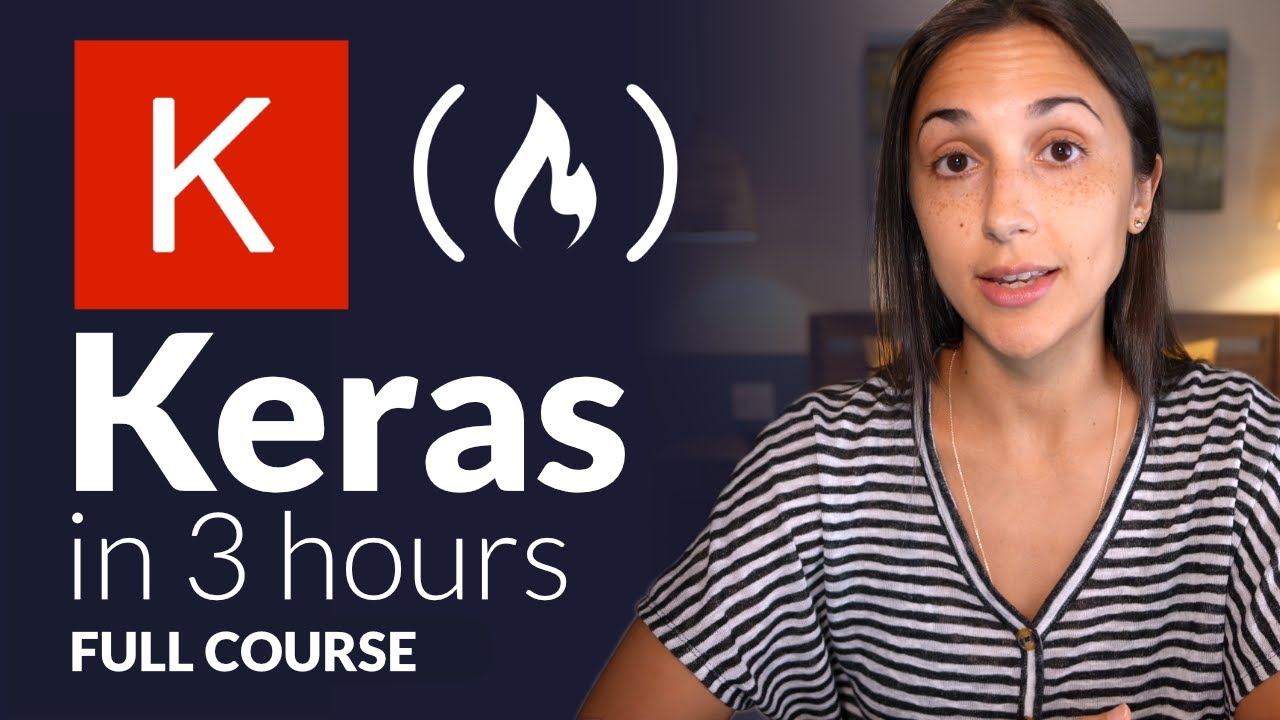# Keras with TensorFlow Course - Python Deep Learning and Neural NetworksKeras with TensorFlow Course - Python Deep Learning and Neural Networks for Beginners Tutorial: How to use Keras, a neural network API written in Python and integrated with TensorFlow. We will learn how to prepare and process data for artificial neural networks, build and train artificial neural networks from scratch, build and train convolutional neural networks (CNNs), implement fine-tuning and transfer learning, and more!

This course will teach you how to use Keras, a neural network API written in Python and integrated with TensorFlow. We will learn how to prepare and process data for artificial neural networks, build and train artificial neural networks from scratch, build and train convolutional neural networks (CNNs), implement fine-tuning and transfer learning, and more!

⭐️🦎 COURSE CONTENTS 🦎⭐️ ⌨️ (00:00:00) Welcome to this course ⌨️ (00:00:16) Keras Course Introduction ⌨️ (00:00:50) Course Prerequisites ⌨️ (00:01:33) DEEPLIZARD Deep Learning Path ⌨️ (00:01:45) Course Resources ⌨️ (00:02:30) About Keras ⌨️ (00:06:41) Keras with TensorFlow - Data Processing for Neural Network Training ⌨️ (00:18:39) Create an Artificial Neural Network with TensorFlow's Keras API ⌨️ (00:24:36) Train an Artificial Neural Network with TensorFlow's Keras API ⌨️ (00:30:07) Build a Validation Set With TensorFlow's Keras API ⌨️ (00:39:28) Neural Network Predictions with TensorFlow's Keras API ⌨️ (00:47:48) Create a Confusion Matrix for Neural Network Predictions ⌨️ (00:52:29) Save and Load a Model with TensorFlow's Keras API ⌨️ (01:01:25) Image Preparation for CNNs with TensorFlow's Keras API ⌨️ (01:19:22) Build and Train a CNN with TensorFlow's Keras API ⌨️ (01:28:42) CNN Predictions with TensorFlow's Keras API ⌨️ (01:37:05) Build a Fine-Tuned Neural Network with TensorFlow's Keras API ⌨️ (01:48:19) Train a Fine-Tuned Neural Network with TensorFlow's Keras API ⌨️ (01:52:39) Predict with a Fine-Tuned Neural Network with TensorFlow's Keras API ⌨️ (01:57:50) MobileNet Image Classification with TensorFlow's Keras API ⌨️ (02:11:18) Process Images for Fine-Tuned MobileNet with TensorFlow's Keras API ⌨️ (02:24:24) Fine-Tuning MobileNet on Custom Data Set with TensorFlow's Keras API ⌨️ (02:38:59) Data Augmentation with TensorFlow' Keras API ⌨️ (02:47:24) Collective Intelligence and the DEEPLIZARD HIVEMIND

## Deep Learning from Scratch and Using Tensorflow in Python

In this article, we will learn how deep learning works and get familiar with its terminology — such as backpropagation and batch size

## PyTorch for Deep Learning | Data Science | Machine Learning | Python

PyTorch for Deep Learning | Data Science | Machine Learning | Python. PyTorch is a library in Python which provides tools to build deep learning models. What python does for programming PyTorch does for deep learning. Python is a very flexible language for programming and just like python, the PyTorch library provides flexible tools for deep learning.

## Machine Learning, Data Science and Deep Learning with Python

Complete hands-on Machine Learning tutorial with Data Science, Tensorflow, Artificial Intelligence, and Neural Networks. Introducing Tensorflow, Using Tensorflow, Introducing Keras, Using Keras, Convolutional Neural Networks (CNNs), Recurrent Neural Networks (RNNs), Learning Deep Learning, Machine Learning with Neural Networks, Deep Learning Tutorial with Python

## Deep Learning Models in Keras - Exploratory Data Analysis (EDA)

Deep learning is one of the most interesting and promising areas of artificial intelligence (AI) and machine learning currently. With great advances in technology and algorithms in recent years, deep learning has opened the door to a new era of AI applications.

## Deep Learning Tutorial with Python | Machine Learning with Neural Networks

In this video, Deep Learning Tutorial with Python | Machine Learning with Neural Networks Explained, Frank Kane helps de-mystify the world of deep learning and artificial neural networks with Python!